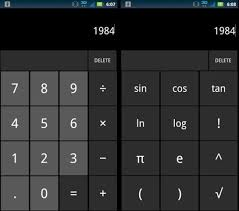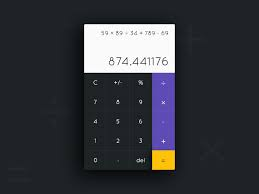# Calculater

982  View(s)
Ratings:
Rate this:

How could I use simultaneously addition subtractions multiple division.

1.##### Re: Calculater

Creating a Calculator for Android devices :

Arithematic operations implemented: +,-,*,/

Boolean operations implemented: and, or, not, xor Primality check, finding mod, exponent, GCD operations have also been implemented.

Design a Interface :

First we will create the GUI of our application coz interface of any application is very major effective part in development.

- grid layout

- TextView

- res/layout```<?xml version="1.0" encoding="utf-8"?> <set xmlns:android="http://schemas.android.com/apk/res/android">
<scale
android:fromXScale="1.0"
android:toXScale="2.0"
android:fromYScale="1.0"
android:toYScale="2.0"
android:duration="50"
android:pivotY="50%"
android:pivotX="50%"
android:repeatCount="1"
android:repeatMode="reverse"/> </set>```